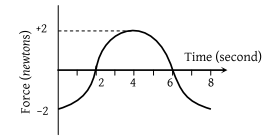NEET Physics Laws of Motion Questions Solved

A force-time graph for a linear motion is shown in figure where the segments are circular. The linear momentum gained between zero and 8 second is(1) $-2\pi \text{\hspace{0.17em}}newton×second$

(2) $Zero\text{\hspace{0.17em}}newton×second$

(3) $+4\pi \text{\hspace{0.17em}}newton×second$

(4) $-6\pi \text{\hspace{0.17em}}newton×second$

Concept Videos :-

#4-Law-of-Inertia
#5-Law-of-Momentum
#6-Law-of-Action-Reaction

Concept Questions :-

Newton laws
Explanation is a part of a Paid Course. To view Explanation Please buy the course.

Difficulty Level: